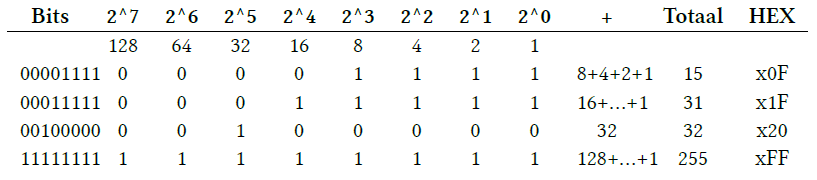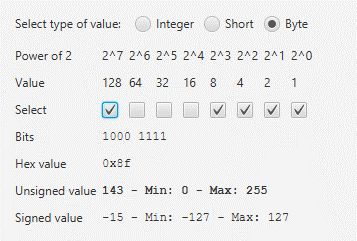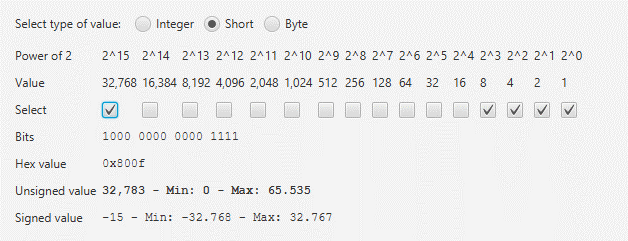# A story of bits, bytes, signed and unsigned

Some time ago there was a question on the Pi4J-forum caused by some confusion about a numeric value handled as a byte which was logged as a negative number -86 instead of the expected value 170. So this is my attempt to try to solve this mystery… ;-)

### The basics

A lot of interaction with the GPIO’s requires some understanding of bits/bytes. So let’s dive into a quick summary.

Very short:

• All logic inside a computers brain is a bit which can be 0 or 1 (off or on).
• When you combine 8 bits, you get a byte.

If you go further in the chain you get this:

• 8 bits = 1 byte
• 1024 bytes = 1 kilobyte
• 1024 kilobytes = 1 megabyte
• 1024 megabytes = 1 gigabyte
• 1024 gigabytes = 1 terabyte

### Convert bits to a numeric and hex value

A combination of multiple bits is converted to a number by using the power of 2.

In everyday life we are used to decimal values where we group everything by 10, 20, 30. In programming, hexadecimal values are used more, and they have the range 0 to 15, which perfectly matches the maximum value of four bits (“1111”). A hex value is written as x0 to xF.

The following table shows all possible combinations of 4 bits ranging from “0000” to “1111”:### Calculate a byte value

A byte consists of 8 bits and has the range of 0x00 (= 0) to 0xFF (= 255).

So we need to extend the table above to have 8 bits. Let’s take a few examples:### Values in Java

Let’s check in Java how values are represented with the following code:

class PrintLimits {
public static void main(String[] args) {
System.out.println("Byte");
System.out.println("    Min: " + Byte.MIN_VALUE);
System.out.println("    Max: " + Byte.MAX_VALUE);

System.out.println("Short");
System.out.println("    Min: " + Short.MIN_VALUE);
System.out.println("    Max: " + Short.MAX_VALUE);

System.out.println("Integer");
System.out.println("    Min: " + Integer.MIN_VALUE);
System.out.println("    Max: " + Integer.MAX_VALUE);

System.out.println("Long");
System.out.println("    Min: " + Long.MIN_VALUE);
System.out.println("    Max: " + Long.MAX_VALUE);
}
}


As a result we get these values:

Byte
Min: -128
Max: 127
Short
Min: -32768
Max: 32767
Integer
Min: -2147483648
Max: 2147483647
Long
Min: -9223372036854775808
Max: 9223372036854775807


Hmm, this is unexpected! A byte has the range of -128 to 127, instead of 0 to 255?!
To understand this, we need to know the difference between signed and unsigned values.

### Signed versus unsigned

When you calculate the byte value to a signed number value, the major bit (the most left one) is handled as an indicator for a negative number (1) or positive number (0), for example 8 bits “1000 1111”:“1000 1111” is both -15 and 143 depending if you handle it as signed or unsigned.

The same goes for a short which consist of two bytes (= 16 bits), for example “1000 0000 0000 1111”:“1000 0000 0000 1111” is both -15 and 32.783 depending if you handle it as signed or unsigned.

### Conclusion

You need to be sure how you handle numeric values when you read them out to not confuse between the signed and unsigned value!

Luckily we have the Byte.toUnsignedInt(b) function, to make sure we are reading out the correct value when logging the values. Here a quick demo with the 170 value from the original Pi4J-forum question:

class HexIntegerToString {
public static void main(String[] args) {
convertByte((byte) 170);
}

private static void convertByte(byte value) {
System.out.println("Byte Unsigned: " + Byte.toUnsignedInt(value) + "\tSigned: " + value);
System.out.println("    Hex value:  0x" + Integer.toHexString(value & 0xFF));
System.out.println("    Binair:     " + Integer.toBinaryString(value & 0xFF));
}
}


Which gives this output:

Byte Unsigned: 170      Signed: -86
Hex value:  0xaa
Binair:     10101010